Next: 3.3.2 Curvature Up: 3.3 Approximations to Geometric Previous: 3.3 Approximations to Geometric

## 3.3.1 Surface Normal

Generally, the normal vector at point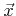on the LS of a smooth function is given by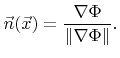(3.21)

At grid points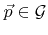the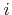-th component of the normal vector can be approximated by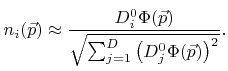(3.22)

Here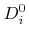is the central difference operator as defined in (3.6). The normal vector for a grid point close to the surfacerepresented by the zero LS is also a good approximation for the normal on the surface for the closest surface point. The closest surface point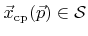of a nearby grid point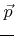can be approximated by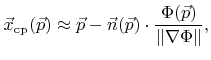(3.23)

if the grid point indicesare equal to the grid point coordinates. Here the last factor corresponds to the approximated signed distance to the surface. For the denominator the same approximation is used as in (3.22).Next: 3.3.2 Curvature Up: 3.3 Approximations to Geometric Previous: 3.3 Approximations to Geometric

Otmar Ertl: Numerical Methods for Topography Simulation